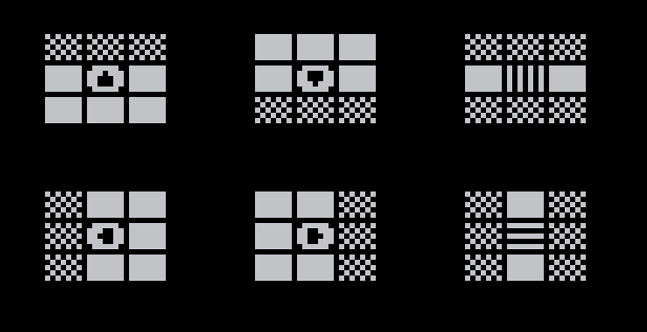A tiny demake of my game Patrick's Cyberpunk Challenge for the TweetTweetJam. The PICO-8 source code fits in two tweets (560 characters).

## Instructions

The object of the game is to move Patrick the Leprechaun around the board and remove all 28 squares. Squares with special symbols will remove extra squares.## Controls

Move Patrick to a square using the arrow keys.

Note that you can (and sometimes must) move diagonally by pressing two arrow keys simultaneously.

## Limitations

• Puzzles might be impossible to solve!
• The game doesn't recognize if you win or lose. If the screen is empty except for Patrick, you win! If you have no legal moves, you lose and must restart the game manually.

## Source code

```poke(24364,3)x="웃"v="▥"h="▤"b={}for i=1,36 do b[i]=i%9<2 and""or"█"end for i in all{"⬆️","➡️",h,"⬅️","⬇️",v,x}do repeat f=1+flr(rnd(28))until b[f]!=""b[f]=i
if(i==x)p=f
end::_::t=btnp cls()for i=0,35 do
k=b[i+1]
?k,i%9*8,6*flr(i/9)+20,k==x and 11 or 7
end b[p]=""q=p
if(t(0))q-=1
if(t(1))q+=1
if(t(2))q-=9
if(t(3))q+=9
if(b[q]and#b[q]>0)p=q t=b[p]
if(t=="⬆️"or t==v)b[p-10]=""b[p-9]=""b[p-8]=""
if(t=="⬇️"or t==v)b[p+10]=""b[p+9]=""b[p+8]=""
if(t=="⬅️"or t==h)b[p-10]=""b[p-1]=""b[p+8]=""
if(t=="➡️"or t==h)b[p+10]=""b[p+1]=""b[p-8]=""
b[p]=x flip()goto _
```
 Status Released Platforms HTML5 Rating Rated 4.5 out of 5 stars(2 total ratings) Author Tobias V. Langhoff Genre Puzzle Made with PICO-8 Tags Demake, Lo-fi, Minimalist Average session A few seconds Inputs Keyboard Accessibility Color-blind friendly, High-contrast, Textless Links Source code